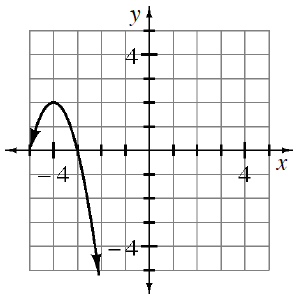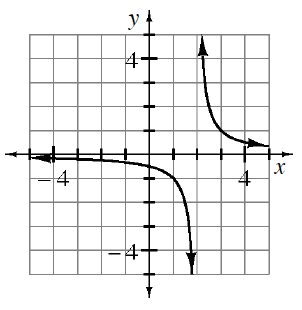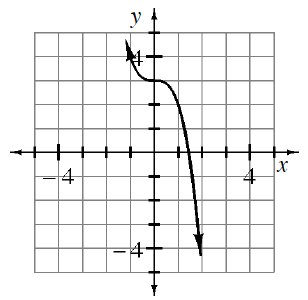### Home > CCA2 > Chapter Ch6 > Lesson 6.1.2 > Problem6-23

6-23.

Find an equation that will generate each graph.

 a.b.c.What kind of graph is this?

What are the x-intercepts and the vertex?

Use the vertex to write equation in graphing form.
Then substitute one of the x-intercepts in for x and y to solve for a.
$y=a(x+4)^2+2$

The parent graph is $\text{ }y=\frac{1}{x}$ and the locator point is $(2,0)$.

See part (a).